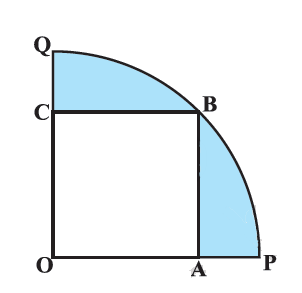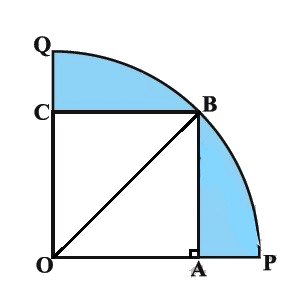# Ex.12.3 Q13 Areas Related to Circles Solution - NCERT Maths Class 10

Go back to  'Ex.12.3'

## Question

In Figure, a square $${OABC}$$ is inscribed in a quadrant $${OPBQ.}$$ If $$OA = \text {20 cm,}$$ find the area of the shaded region.

(Use $$\pi =3.14$$ )Video Solution
Areas Related To Circles
Ex 12.3 | Question 13

## Text Solution

What is known?

A square $${OABC}$$ is inscribed in a quadrant $$OPBQ, OA =\text{ 20 cm.}$$

What is unknown?

Reasoning:

Visually from the figure it is clear that

Area of the shaded region $$=$$ Area of quadrant $${OPBQ}\, -$$ Area of square $${OABC}$$

Since side of the square $$OA =\text{ 20 cm}$$

$$\rm{OB =}$$ Radius $$=$$ Diagonal of the square $${OABC}$$

Using formula for

Area of sector of angle \begin{align}\theta = \frac{\theta }{{{{360}^\circ }}} \times \pi {r^2}\end{align}

With $$\theta = 90^\circ$$ and $$r = OB$$

We can find the area of the quadrant $${OPBQ.}$$

Steps:Join $${OB.}$$

We know $$\Delta {OBA}$$ is a right angaled triangle, a $$\angle {OAB} \,= {90^ \circ }$$ (angle of a square)

$$\therefore\;$$Using Pythagoras theorem

\begin{align} \therefore\quad {{OB}^{2}}&={{OA}^{2}}+A{{B}^{2}} \\ &={{(20)}^{2}}+{{(20)}^{2}} \\ {DB}&=\sqrt{2{{(20)}^{2}}} \\ &=20\sqrt{2}\,\rm{cm}\end{align}

Therefore,radius of the quadrant,\begin{align}r = OB = 20\sqrt 2 cm\end{align}

\begin{align}&= \frac{{{{90}^\circ }}}{{{{360}^\circ }}} \times \pi {r^2}\\ &= \frac{1}{4} \times 3.14 \times {(20\sqrt 2 )^2}\\ &= \frac{1}{4} \times 3.14 \times {400} \times 2\\&= 628{\text{ cm}^2}\end{align}

Area of square OABC

\begin{align} &= {\left( {side} \right)^2}\\&= {\left( {OA} \right)^2}\\&= {\left( {20cm} \right)^2}\\&= 400c{m^2}\end{align}

Area of shaded region $$=$$ Area of quadrant $${OPBQ}\;-$$ Area of square $${OABC}$$

\begin{align}&= {628 - 400{\text{ cm}^2}}\\&= {228{\text{ cm}^2}}\end{align}

Learn from the best math teachers and top your exams

• Live one on one classroom and doubt clearing
• Practice worksheets in and after class for conceptual clarity
• Personalized curriculum to keep up with school